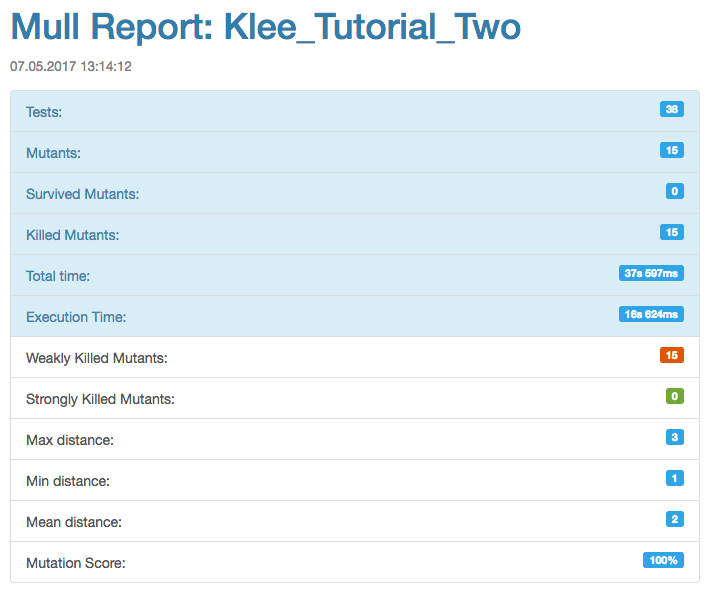Comparison between Mull and Klee and finding possible ways of collaboration between these two tools has been our long-standing goal: Mull#121: Research is needed: compare Mull with Klee. This post is a report about the first experiment that we did with Mull and Klee:

1. Follow through Klee’s Tutorial Two: generate Klee’s tests for simple regular expression matching function (this function is a system under test in that tutorial).
2. Using `ktest-tool`, manually create a test suite based on Google Test out of Klee’s binary test files generated at step 1.
3. Run Mull against a manually created test suite and observe the results.
4. Analyze the results, do fixes and write more tests if necessary to achieve 100% mutation testing coverage.
5. Make observations.
6. Conclusion.

TL;DR This is a long post which requires following the code examples and the screenshots. Here are the results if you want to skip the content:

• For tutorial Two we were only able to achieve mutation score of 87% having only the tests generated by Klee. Adding redundancy of more inputs and generating more tests with Klee didn’t improve the result over this limit of 87%.
• Along the process, these tests were converted by us manually and resulting test suite showed significant redundancy. The same mutation score of 87% and finally a mutation score of 100% could be achieved by a smaller number of tests.
• Our knowledge of Klee is very limited. We only followed tutorials One and Two and we are not aware of various configuration options that Klee allows to experiment with. We do understand that we could get different results if we would know Klee better.
• This simple regex matching function as a system under test is not the best option for a Klee tutorial. As we looked at the tests generated by Klee we saw that positive or negative matches for some of the inputs were not consistent with each other. The function is a simple function taken from a book so it is fine that it does not produce what we would expect from a real production regex library, however, this complicated our analysis a bit because we didn’t have a clear mental model but only a combination of the tests that we treated as a spec that we should have followed.

### Step 1: Generating Klee’s test for a simple regex library

Here is a source code which is a system under test of Tutorial Two. To make the code more readable for a further analysis the braces are added so that:

``````if (condition)
return value;
``````

becomes:

``````if (condition) {
return value;
}
``````

The code:

``````/*
* Simple regular expression matching.
*
* From:
*   The Practice of Programming
*   Brian W. Kernighan, Rob Pike
*
*/

static int matchhere(char*,char*);

static int matchstar(int c, char *re, char *text) {
do {
if (matchhere(re, text)) {
return 1;
}
} while (*text != '\0' && (*text++ == c || c== '.'));
return 0;
}

static int matchhere(char *re, char *text) {
if (re == '\0') {
return 0;
}

if (re == '*') {
return matchstar(re, re+2, text);
}

if (re == '\$' && re=='\0') {
return *text == '\0';
}

if (*text != '\0' && (re=='.' || re==*text)) {
return matchhere(re+1, text+1);
}

return 0;
}

int match(char *re, char *text) {
if (re == '^') {
return matchhere(re+1, text);
}

do {
if (matchhere(re, text)) {
return 1;
}
} while (*text++ != '\0');

return 0;
}
``````

We follow the advice from the tutorial to use `klee_assume` to teach Klee that we only want to work with zero-terminated strings otherwise Klee produces the test cases with errors that we don’t want to deal with within a scope of this post:

``````// The size of the buffer to test with.
#define SIZE 7

int main() {
// The input regular expression.
char re[SIZE];

// Make the input symbolic.
klee_make_symbolic(re, sizeof re, "re");
klee_assume(re[SIZE - 1] == '\0');

// Try to match against a constant string "hello".
match(re, "hello");

return 0;
}
``````

When we run Klee on this code, we get the following result:

``````klee@0265a1bae7c7:~/klee_src/examples/regexp\$ clang -I ../../include -emit-llvm -c -g Regexp.c
klee@0265a1bae7c7:~/klee_src/examples/regexp\$ klee --only-output-states-covering-new Regexp.bc
KLEE: output directory is "/home/klee/klee_src/examples/regexp/klee-out-3"
KLEE: Using STP solver backend

KLEE: done: total instructions = 4016685
KLEE: done: completed paths = 5895
KLEE: done: generated tests = 14
``````

### Step 2: Manually creating a test suite from Klee’s auto-generated tests

This is an example of the first auto-generated test:

``````\$ ktest-tool test000001.ktest
ktest file : 'test000001.ktest'
args       : ['Regexp.bc']
num objects: 1
object    0: name: b're'
object    0: size: 7
object    0: data: b'^\x00\x00\x00\x00\x00\x00'
``````

This is what we create manually out of this auto-generated test:

``````TEST(KleeTest, hello_test000001) {
char re[] = "^";

int res = match(re, (char *)"hello");

ASSERT_EQ(res, 0);
}
``````

We follow this procedure for all of the 14 tests and get the following test suite:

Manually-created test suite
```TEST(KleeTest, hello_test000001) {
char re[] = "^";

int res = match(re, (char *)"hello");

ASSERT_EQ(res, 0);
}

TEST(KleeTest, hello_test000002) {
char re[] = "^\$";

int res = match(re, (char *)"hello");

ASSERT_EQ(res, 0);
}

TEST(KleeTest, hello_test000003) {
char re[] = "^\$\x01";

int res = match(re, (char *)"hello");

ASSERT_EQ(res, 0);
}

TEST(KleeTest, hello_test000004) {
char re[] = "^\x01";

int res = match(re, (char *)"hello");

ASSERT_EQ(res, 0);
}

TEST(KleeTest, hello_test000005) {
char re[] = "^.";

int res = match(re, (char *)"hello");

ASSERT_EQ(res, 0);
}

TEST(KleeTest, hello_test000006) {
char re[] = "^\x01*";

int res = match(re, (char *)"hello");

ASSERT_EQ(res, 0);
}

TEST(KleeTest, hello_test000007) {
char re[] = "^h";

int res = match(re, (char *)"hello");

ASSERT_EQ(res, 0);
}

TEST(KleeTest, hello_test000008) {
char re[] = "";

int res = match(re, (char *)"hello");

ASSERT_EQ(res, 0);
}

TEST(KleeTest, hello_test000009) {
char re[] = "^.*";

int res = match(re, (char *)"hello");

ASSERT_EQ(res, 0);
}

TEST(KleeTest, hello_test000010) {
char re[] = "\$";

int res = match(re, (char *)"hello");

ASSERT_EQ(res, 1);
}

TEST(KleeTest, hello_test000011) {
char re[] = "\$\x01";

int res = match(re, (char *)"hello");

ASSERT_EQ(res, 0);
}

TEST(KleeTest, hello_test000012) {
char re[] = "^.*\$";

int res = match(re, (char *)"hello");

ASSERT_EQ(res, 1);
}

TEST(KleeTest, hello_test000013) {
char re[] = "e";

int res = match(re, (char *)"hello");

ASSERT_EQ(res, 0);
}

TEST(KleeTest, hello_test000014) {
char re[] = ".*";

int res = match(re, (char *)"hello");

ASSERT_EQ(res, 0);
}
```

### Step 3: Running Mull on a manually-created test suite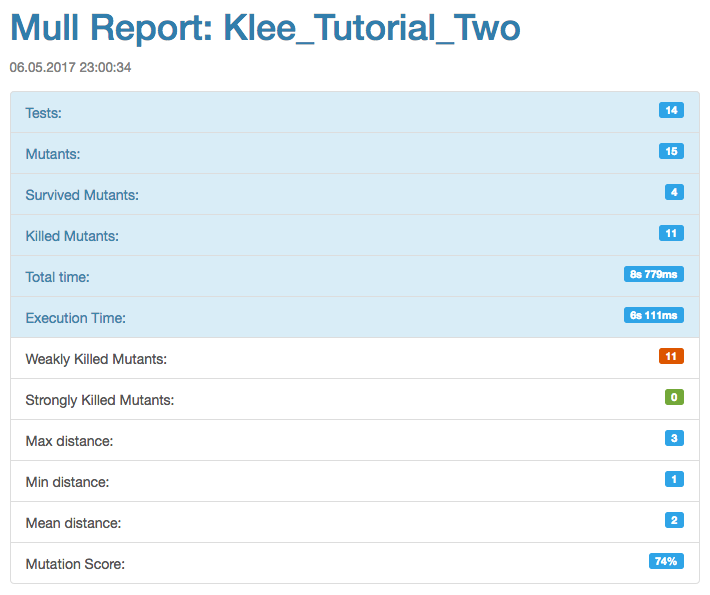The legend below helps to interpret the numbers of this report.

Mull: legend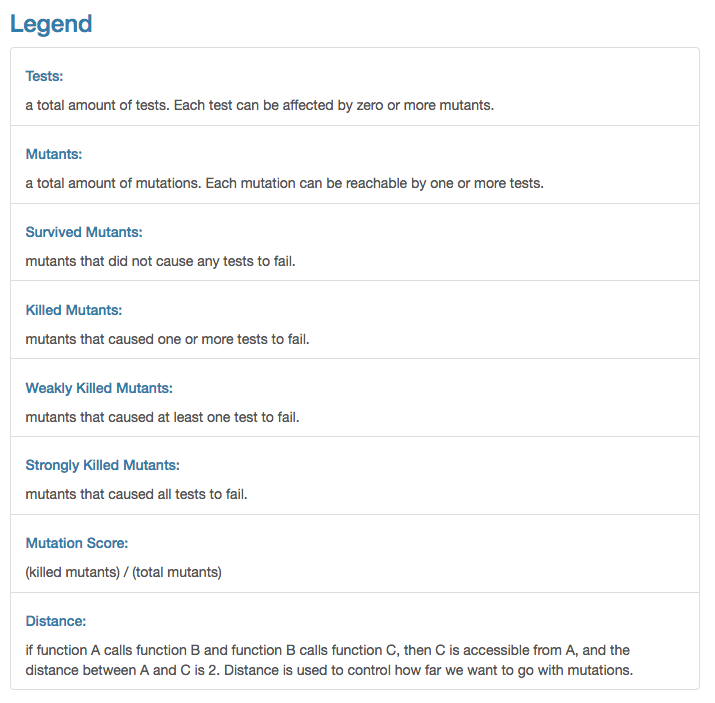### Step 4a: Initial analysis

When we ran Mull on these 14 tests generated by Klee we expected to have 100% mutation score for this test suite because we thought that Klee would have all possible inputs created for the function `match` so that all mutants would be killed by those 14 tests.

Instead, we see that mutation score is 74%: we see that 4 mutants survived which means that these 4 mutations “did not cause any tests to fail” according to the legend above.

Mutation #1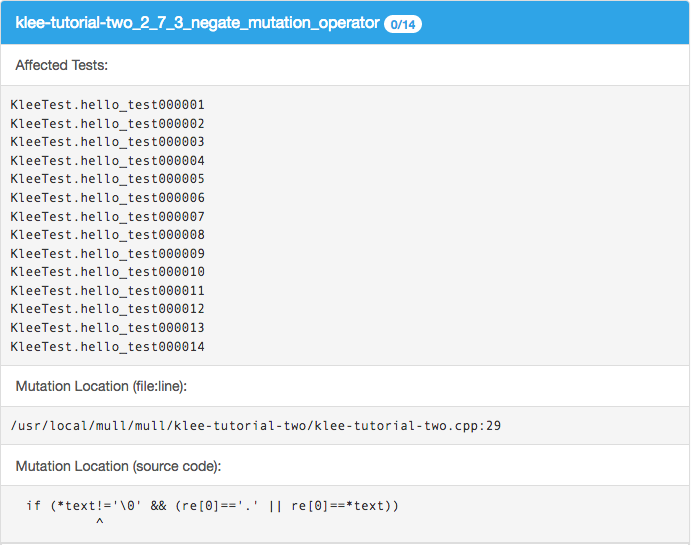Mutation #2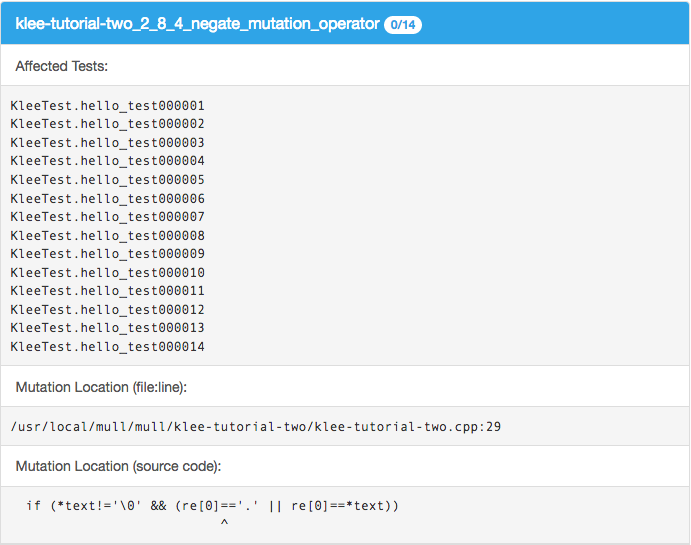Mutation #3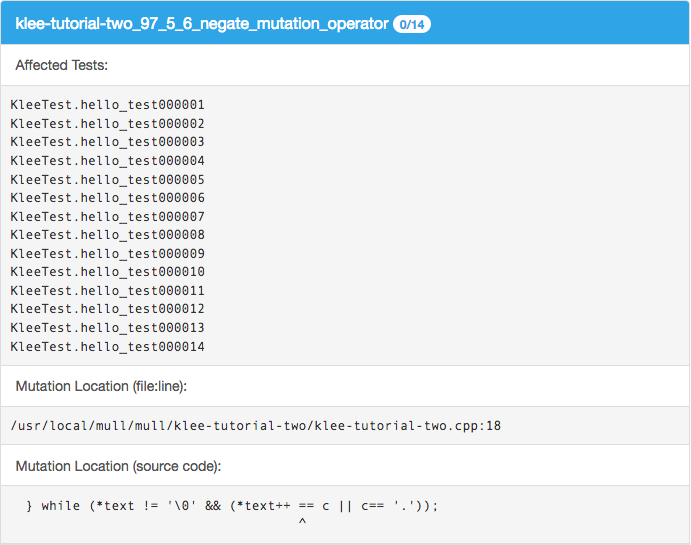Mutation #4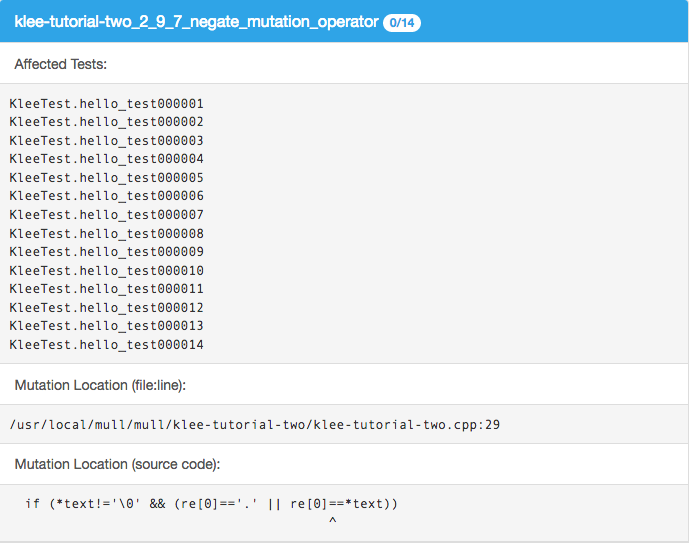Let’s consider mutation #1. Indeed if we manually replace `*text != '\0'` with `*text == '\0'` and run the test suite manually, we see that no tests fail. If we spend a bit more time analyzing this code and especially step through it with a debugger we can quickly come to a hypothesis that we are just missing some tests: Klee only generated those 14 tests for the string `"hello"` while mutation #1 seems to be lacking a test which involves an input of one-symbol string `"h"`.

Let’s run Klee again on the same code but with input “h” instead of “hello”:

### Step 4b: Adding more test scenarios with Klee (“h” instead of “hello”)

Tests for "h" input
```// The size of the buffer to test with.
#define SIZE 7

int main() {
// The input regular expression.
char re[SIZE];

// Make the input symbolic.
klee_make_symbolic(re, sizeof re, "re");
klee_assume(re[SIZE - 1] == '\0');

match(re, "h");

return 0;
}

\$ klee --only-output-states-covering-new Regexp.bc
KLEE: output directory is "/home/klee/klee_src/examples/regexp/klee-out-5"
KLEE: Using STP solver backend

KLEE: done: total instructions = 20417
KLEE: done: completed paths = 166
KLEE: done: generated tests = 13

#pragma mark - Tests: "h" group

TEST(KleeTest, h_test000001) {
char re[] = "^";

int res = match(re, (char *)"h");

ASSERT_EQ(res, 0);
}

TEST(KleeTest, h_test000002) {
char re[] = "";

int res = match(re, (char *)"h");

ASSERT_EQ(res, 0);
}

TEST(KleeTest, h_test000003) {
char re[] = "^\$";

int res = match(re, (char *)"h");

ASSERT_EQ(res, 0);
}

TEST(KleeTest, h_test000004) {
char re[] = "^\x01";

int res = match(re, (char *)"h");

ASSERT_EQ(res, 0);
}

TEST(KleeTest, h_test000005) {
char re[] = "^\$\x01";

int res = match(re, (char *)"h");

ASSERT_EQ(res, 0);
}

TEST(KleeTest, h_test000006) {
char re[] = "^.";

int res = match(re, (char *)"h");

ASSERT_EQ(res, 0);
}

TEST(KleeTest, h_test000007) {
char re[] = "^\x01*";

int res = match(re, (char *)"h");

ASSERT_EQ(res, 0);
}

TEST(KleeTest, h_test000008) {
char re[] = "^h";

int res = match(re, (char *)"h");

ASSERT_EQ(res, 0);
}

TEST(KleeTest, h_test000009) {
char re[] = "^.\x01";

int res = match(re, (char *)"h");

ASSERT_EQ(res, 0);
}

TEST(KleeTest, h_test000010) {
char re[] = "^.*";

int res = match(re, (char *)"h");

ASSERT_EQ(res, 0);
}

TEST(KleeTest, h_test000011) {
char re[] = "\$";

int res = match(re, (char *)"h");

ASSERT_EQ(res, 1);
}

TEST(KleeTest, h_test000012) {
char re[] = "^.\x01*\$";

int res = match(re, (char *)"h");

ASSERT_EQ(res, 1);
}

TEST(KleeTest, h_test000013) {
char re[] = ".*";

int res = match(re, (char *)"h");

ASSERT_EQ(res, 0);
}
```

When we add these tests to the test suite and run Mull again, we get a different report: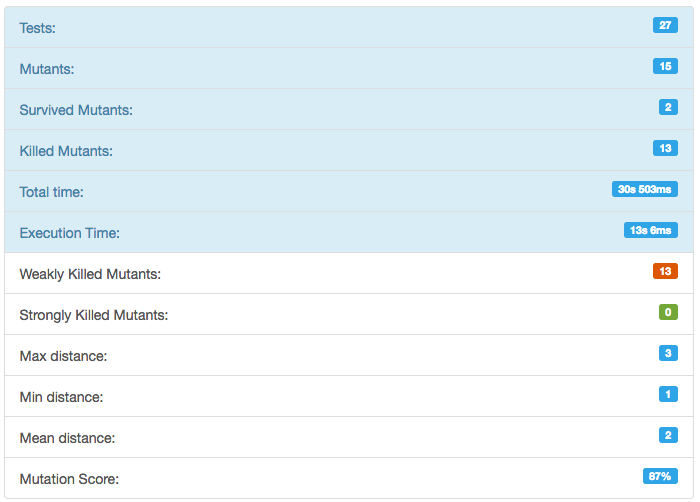Mutation #1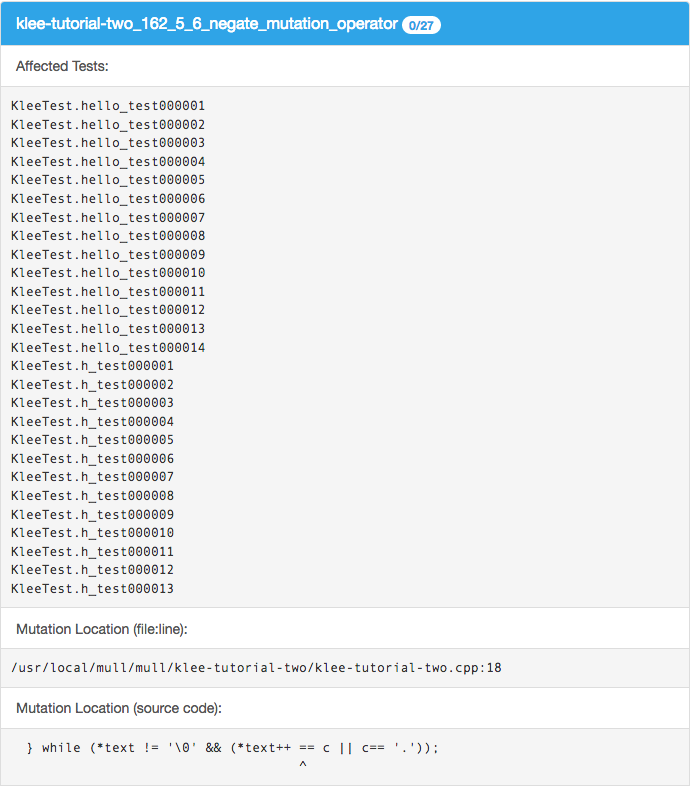Mutation #2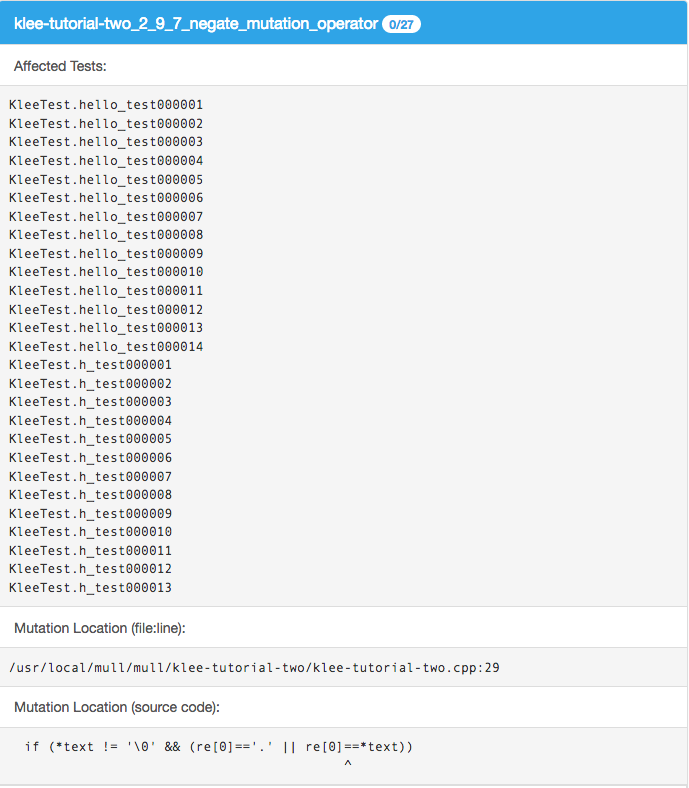We see that newly generated test cases killed 2 of 4 mutations that we had at the step 4a. At this point, we have the tests that Klee generated for “hello” and “h” inputs. Let’s consider the third obvious case: empty string `""`, maybe it will kill either or both of these remaining 2 mutants.

### Step 4c: Adding more test scenarios with Klee (“” instead of “hello”)

Tests for "" input
```// The size of the buffer to test with.
#define SIZE 7

int main() {
// The input regular expression.
char re[SIZE];

// Make the input symbolic.
klee_make_symbolic(re, sizeof re, "re");
klee_assume(re[SIZE - 1] == '\0');

match(re, "");

return 0;
}

\$ klee --only-output-states-covering-new Regexp.bc
KLEE: output directory is "/home/klee/klee_src/examples/regexp/klee-out-4"
KLEE: Using STP solver backend

KLEE: done: total instructions = 1034
KLEE: done: completed paths = 24
KLEE: done: generated tests = 9

#pragma mark - Tests: "" group

TEST(KleeTest, empty_test000001) {
char re[] = "^";

int res = match(re, (char *)"");

ASSERT_EQ(res, 0);
}

TEST(KleeTest, empty_test000002) {
char re[] = "";

int res = match(re, (char *)"");

ASSERT_EQ(res, 0);
}

TEST(KleeTest, empty_test000003) {
char re[] = "^\x01";

int res = match(re, (char *)"");

ASSERT_EQ(res, 0);
}

TEST(KleeTest, empty_test000004) {
char re[] = "\x01";

int res = match(re, (char *)"");

ASSERT_EQ(res, 0);
}

TEST(KleeTest, empty_test000005) {
char re[] = "\$";

int res = match(re, (char *)"");

ASSERT_EQ(res, 1);
}

TEST(KleeTest, empty_test000006) {
char re[] = "\$\x01";

int res = match(re, (char *)"");

ASSERT_EQ(res, 0);
}

TEST(KleeTest, empty_test000007) {
char re[] = "^\x01*";

int res = match(re, (char *)"");

ASSERT_EQ(res, 0);
}

TEST(KleeTest, empty_test000008) {
char re[] = "\x01*";

int res = match(re, (char *)"");

ASSERT_EQ(res, 0);
}

TEST(KleeTest, empty_test000009) {
char re[] = "\x01*\$";

int res = match(re, (char *)"");

ASSERT_EQ(res, 1);
}
```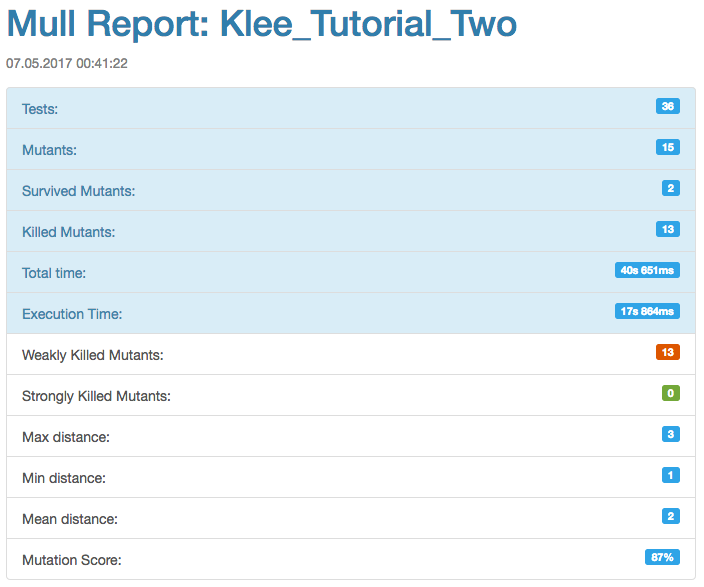Mutation #1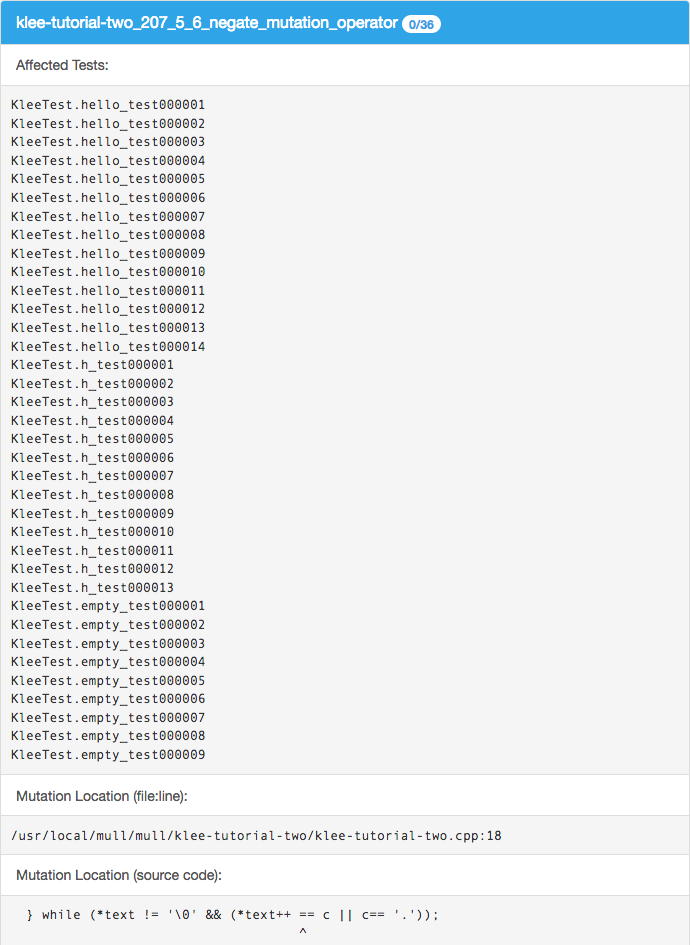Mutation #2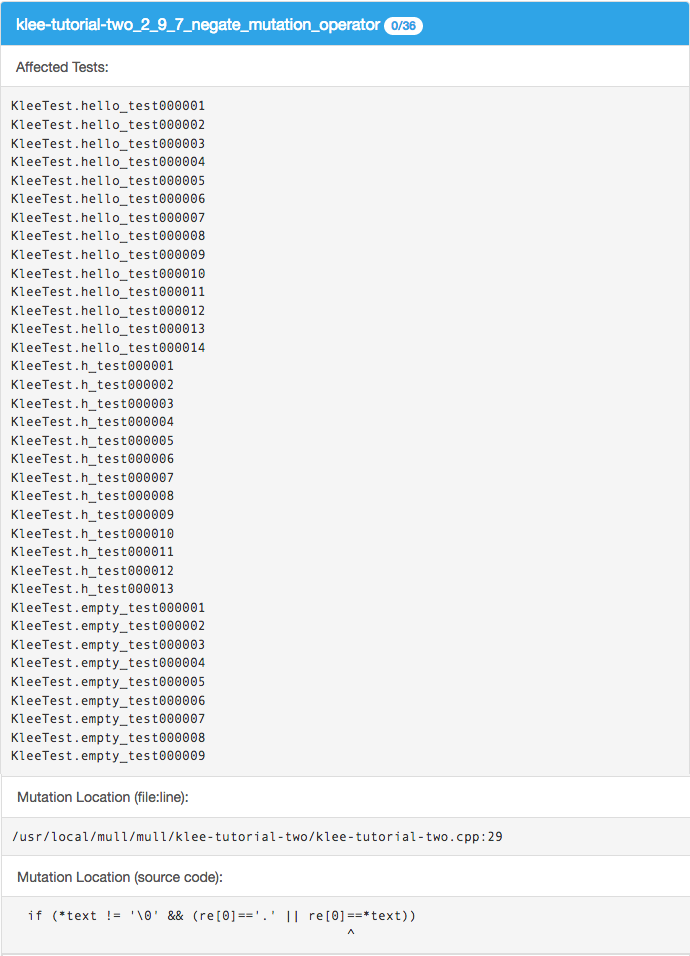We see that tests generated for “” input didn’t change the mutation score and we still have the same 2 mutations from step 4b - they are still not killed by any of 36 tests.

At this point we are running out of options: it is not clear what input we can give Klee to generate tests so that we could have 100% mutation score. With a great redundancy of 36 tests we still have 2 mutants that survive.

### Step 4d: Writing missing tests manually using human brain power

After doing a simple research on mutation #1 and mutation #2 and using debugger to step through their sections of code, the following tests are enough to kill both mutations.

Test that kills Mutation #1
```TEST(KleeTest, kill_Mutation1) {
char re[] = "^1*\$";

int res = match(re, (char *)"1111");

ASSERT_EQ(res, 1);
}
```
Test that kills Mutation #2
```TEST(KleeTest, killMutation2) {
char re[] = "^h\$";

int res = match(re, (char *)"h");

ASSERT_EQ(res, 1);
}
```

A careful reader may ask: why didn’t we use Klee to generate the inputs for a string that has repeating symbols to kill the mutation #1? Indeed, we used Klee to generate another 10 tests for the input “hhh” but none of those tests killed this mutation.

### Step 5a: Observations about redundancy

Observation 1. After step 4a with 4 mutations we went with step 4b where Klee generated 13 tests for input `"h"` which killed 2 mutations of 4. Instead, only one test can be written which kills those 2 mutations. This test can be derived from looking at those mutations and stepping through their critical code with a debugger.

```TEST(KleeTest, killFirstTwoOfFourMutations) {
char re[] = "^.\$";

int res = match(re, (char *)"h");

ASSERT_EQ(res, 1);
}
```

Observation 2. Step 4b killed 2 mutations. Out of all 13 generated tests for `"h"` input, only this test actually killed both mutations:

```if (*text != '\0' && (re=='.' || re==*text))
^
```

and

```if (*text != '\0' && (re=='.' || re==*text))
^
```

The test:

```TEST(KleeTest, h_test000012) {
char re[] = "^.\x01*\$";

int res = match(re, (char *)"h");

ASSERT_EQ(res, 1);
}
```

### Step 5b: Observation about weird matches

Example 1.

The function does not match “^” symbol on all inputs:

``````TEST(KleeTest, empty_test000001) {
char re[] = "^";

int res = match(re, (char *)"");

ASSERT_EQ(res, 0);
}

TEST(KleeTest, hello_test000001) {
char re[] = "^";

int res = match(re, (char *)"hello");

ASSERT_EQ(res, 0);
}
``````

but it does match “\$” symbol:

``````TEST(KleeTest, hello_test000010) {
char re[] = "\$";

int res = match(re, (char *)"hello");

ASSERT_EQ(res, 1);
}

TEST(KleeTest, h_test000011) {
char re[] = "\$";

int res = match(re, (char *)"h");

ASSERT_EQ(res, 1);
}
``````

Example 2.

For the same reason as in Example 1, the function does match this line:

``````TEST(KleeTest, empty_test000009) {
char re[] = "\x01*\$";

int res = match(re, (char *)"");

ASSERT_EQ(res, 1);
}
``````

But does not match on this line:

``````TEST(KleeTest, hello_test000006) {
char re[] = "^\x01*";

int res = match(re, (char *)"hello");

ASSERT_EQ(res, 0);
}
``````

### Conslusion

The major results and conclusions can be found in TL;DR at the beginning of this post. Here are a few more:

1. We are wondering why Klee didn’t generate the test cases to kill the last two mutations. We assume that probably this goes about some details of Klee configuration that we are not aware of. Maybe using some other solver backend instead of `KLEE: Using STP solver backend` we could have these needed tests generated.

2. One thing we are definitely missing in Klee’s toolchain is an option to auto-generate human-readable tests so that a human could inspect Klee’s products much easier. For this post we had to use `ktest-tool` manually for about 50 times and do this job of a test case generation manually which was tedious and of course error-prone. We think that for C and C++ languages Google Test would be a great default option for this feature. Of course, we also mean a bulk generation of a whole test suite for a whole `klee-out` folder as well as one test case generation for a one `.ktest` file.

3. We are not aware if Klee would allow us to make both inputs symbolic so that it executed both `re` and input string parameters so that we did not have to substitute “hello” -> “h” -> “” -> “hhh” inputs manually by hands. However, this goes beyond a scope of this post and a content of Tutorial Two.

This is a final report with redundancy of the tests auto-generated for `"hello"`, `"h"` and `""` strings and two tests that we wrote to kill the last two mutations.

Final report